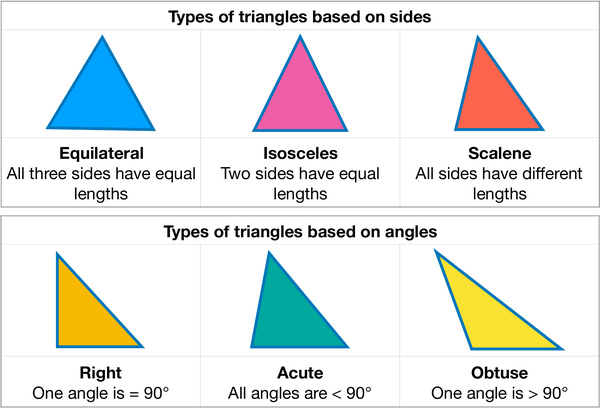# Different Types of Triangles: Equilateral, Isosceles, Scalene, Right, Acute, and Obtuse TrianglesThis sheet presents different types of triangles based on sides (Equilateral, Isosceles, and Scalene) and angles (Right, Acute, and Obtuse).

Equilateral triangles: Equilateral triangles have three equal sides and three equal angles. All angles in an equilateral triangle are 60 degrees. They are often used in geometry because they are symmetrical and have some interesting properties.

Isosceles triangles: Isosceles triangles have two equal sides and two equal angles. The third side and angle may be different. The two equal angles are opposite the two equal sides. Isosceles triangles can be acute, right or obtuse.

Scalene triangles: Scalene triangles have three different sides and three different angles. None of the angles or sides are equal. Because scalene triangles are irregular, they can have a variety of angles, ranging from acute to obtuse.

Right triangles: Right triangles have one angle that measures 90 degrees, which is called a right angle. The side opposite the right angle is called the hypotenuse, and the other two sides are called the legs. The Pythagorean theorem, which states that the square of the hypotenuse is equal to the sum of the squares of the legs, is often used to solve problems involving right triangles.

Acute triangles: Acute triangles have three angles that are all less than 90 degrees. This means that all three sides of an acute triangle are acute angles.

Obtuse triangles: Obtuse triangles have one angle that is greater than 90 degrees, which is called an obtuse angle. The other two angles are acute angles. In an obtuse triangle, the side opposite the obtuse angle is the longest side.

Understanding the different types of triangles is an important part of learning geometry. It can help kids identify and classify shapes correctly. This can also be useful in geometry, where kids may need to distinguish between different types of triangles for problem-solving purposes. Each type of triangle has its own unique properties, such as angle measurements or side lengths. Understanding these properties can help kids solve problems related to triangles, such as finding missing angles or sides.

When discussing shapes or solving problems with others, it's important to use accurate terminology. By knowing the names and properties of different types of triangles, kids can communicate more effectively with others. Triangles are found in many real-world applications, such as architecture, engineering, and physics. Understanding the different types of triangles and their properties can help kids in these fields by allowing them to make accurate measurements and calculations.